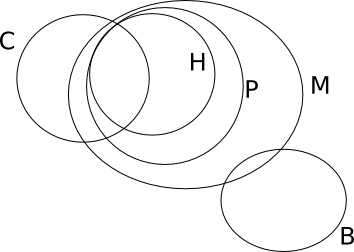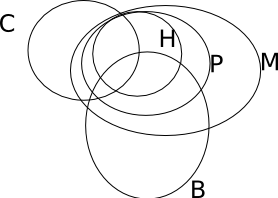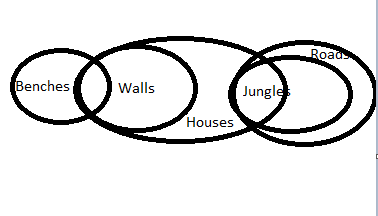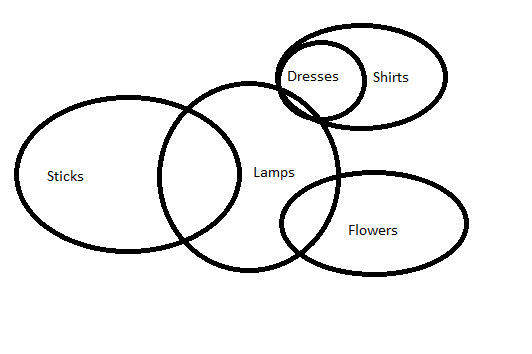# Syllogism Questions and Answers for SBI Clerk

1
9294

Syllogism is the of the most important topic, every year around 5 questions will be asked from this topic, Syllogism questions and answers can be solved by forming diagrams, and the maximum overlaps, minimum overlaps, would help you to get to know which given statement is possible.

## Syllogism Questions and Answers for SBI Clerk:

You can download syllogism questions PDF or you can directly go through the questions given below.

For more questions visit SBI Clerk Previous question papers (solved)

Instructions (1-5):

In each of the questions below are given four statements followed by four conclusions numbered I, II, III & IV. You have to take the given statements to be true even if they seem to be at given conclusions logically follows from the given statements disregarding commonly known facts.

Question 1: Statements:
All cups are bottles.
Some bottles are jugs.
No jug is plate.
Some plates are tables.

Conclusions:
I. Some tables are bottles.
II. Some plates are cups.
III. No table is bottle.
IV. Some jugs are cups.

a) Only I follows
b) Only II follows
c) Only III follows
d) Only IV follows
e)Only either I or III follows

Question 2:

Statements:
Some chairs are handles.
All handles are pots.
All pots are mats.
Some mats are buses.

Conclusions:
I. Some buses are handles.
II. Some mats are chairs.
III. No bus is handle.
IV. Some mats are handles.

a) Only I, II and IV follow
b) Only II, III and IV follow
c) Only either I or III and II follow
d) Only either I or III and IV follow
e)Only either I or III and II and IV follow

Question 3:

Statements:
All birds are horses.
All horses are tigers.
Some tigers are lions.
Some lions are monkeys.

Conclusions:
I. Some tigers are horses.
II. Some monkeys are birds.
III. Some tigers are birds.
IV. Some monkeys are horses.

a) Only I and III follow
b) Only I, II and III follow
c) Only II, III and IV follow
d) All I, II, III and IV follow
e)None of these

Question 4:

Statements:
Some benches are walls.
All walls are houses.
Some houses are jungles.

Conclusions:
II. Some jungles are walls.
III. Some houses are benches.

a) Only land II follow
b) Only I and III follow
c) Only III and IV follow
d) Only II, III and IV follow
e)None of these

Question 5:

Statements:
Some sticks are lamps.
Some flowers are lamps.
Some lamps are dresses.
All dresses are shirts.

Conclusions:
I. Some shirts are sticks.
II. Some shirts are flowers.
III. Some flowers are sticks.
IV. Some dresses are sticks.

a) None follows
b) Only I follows
c) Only II follows
d) Only III follows
e)Only IV follows

Instructions (6 to 7) :

In each of the questions below, two/three statements are given followed by conclusions/ group of conclusions numbered I and II. You have to assume all the statements to be true even if they seem to be at variance with the commonly known facts and then decide which of the given two conclusions logically follows from the information given in the statements.

Question 6:

Statements:
Some squares are circles.
No circle is a triangle.
No line is a square.

Conclusions:
I. All squares can never be triangles.
II. Some lines are circles.

a) Only conclusion I follows.
b) Only conclusion II follows.
c) Either conclusion I or conclusion II follows.
d) Neither conclusion I nor conclusion II follows.
e)Both conclusion I and II follow.

Question 7:

Statements:
Some squares are circles.
No circle is a triangle.
No line is a square.

Conclusions:
I. No triangle is a square.
II. No line is a circle

a) Only conclusion I follows.
b) Only conclusion II follows.
c) Either conclusion I or conclusion II follows.
d) Neither conclusion I nor conclusion II follows.
e)Both conclusion I and II follow.

Question 8:

Statements:
All songs are poems.
All poems are rhymes.
No rhyme is paragraph.

Conclusions:
I. No Song is a paragraph.
II. No poem is a paragraph.

a) Only conclusion I follows.
b) Only conclusion II follows.
c) Either conclusion I or conclusion II follows.
d) Neither conclusion I nor conclusion II follows.
e)Both conclusion I and II follow.

Question 9:

Statements:
All songs are poems.
All poems are rhymes.
No rhyme is paragraph.

Conclusions:
I. All rhymes are poems.
II. All Songs are rhymes.

a) Only conclusion I follows.
b) Only conclusion II follows.
c) Either conclusion I or conclusion II follows.
d) Neither conclusion I nor conclusion II follows.
e)Both conclusion I and II follow.

Question 10:

Statements:
Some dews are drops.
All drops are stones.

Conclusions:
I. At least some dews are stones.
II. At least some stones are drops.

a) Only conclusion I follows.
b) Only conclusion II follows.
c) Either conclusion I or conclusion II follows.
d) Neither conclusion I nor conclusion II follows.
e)Both conclusion I and II follow.

Solutions 1 to 5 :

The conclusions talk about a relation between tables and bottles. Let’s bring a relation between these two using the given statements.
Some bottles are jugs and no jug is a plate => some bottles can be plates or no bottle is a plate
Some plates are tables => some bottles can be plates or no bottle is a plate.  — (1)
All cups are bottles and some bottles are jugs => Nothing about cups and jugs can be derived from this. Since we cannot derive a conclusion between cups and jugs, it is not possible to derive a conclusion between cups and plates as well. Hence option E, which says that some bottles can be plates or no bottle is a plate is the answer.

The following are the two possibilities:So, either I or III and II and IV follow.

Birds is a subset of Horses, which is a subset of Tigers. So, conclusions I and III follow. But, conclusions II and IV need not follow.

Based on the following Venn diagram, only conclusions 3 and 4 are implied.Based on the Venn diagram, none of the conclusions are implied by the statements.

Solutions ( 6 to 10 ):

Some squares are circles and no circle is a triangle => Some squares are not triangles => Conclusion 1 follows No line is a square => No relation can be brought between line and circle => Conclusion 2 does not follow.

Some squares are circles and no circle is a triangle => Some squares are not triangles => Some squares being triangles is a possibility => Conclusion 1 does not follow No line is a square and some square are circle => Some lines being circles is a possibility => Conclusion 2 does not follow

All songs are poems and all poems are rhymes => All songs are rhymes
No rhyme is a paragraph => No song is a paragraph => Conclusion 1 follows
All poems are rhymes and no rhyme is a paragraph => No poem is a paragraph => Conclusion 2 follows